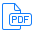# Trapezoidal Generalization over Linear Constraints## D. Greve, A. Gacek

### 15th International Workshop on the ACL2 Theorem Prover and Its Applications, November 2018

We are developing a model-based fuzzing framework that employs mathematical models of system behavior to guide the fuzzing process. Whereas traditional fuzzing frameworks generate tests randomly, a model-based framework can deduce tests from a behavioral model using a constraint solver. Because the state space being explored by the fuzzer is often large, the rapid generation of test vectors is crucial. The need to generate tests quickly, however, is antithetical to the use of a constraint solver. Our solution to this problem is to use the constraint solver to generate an initial solution, to generalize that solution relative to the system model, and then to perform rapid, repeated, randomized sampling of the generalized solution space to generate fuzzing tests. Crucial to the success of this endeavor is a generalization procedure with reasonable size and performance costs that produces generalized solution spaces that can be sampled efficiently. This paper describes a generalization technique for logical formulae expressed in terms of Boolean combinations of linear constraints that meets the unique performance requirements of model-based fuzzing. The technique represents generalizations using trapezoidal solution sets consisting of ordered, hierarchical conjunctions of linear constraints that are more expressive than simple intervals but are more efficient to manipulate and sample than generic polytopes. Supporting materials contain an ACL2 proof that verifies the correctness of a low-level implementation of the generalization algorithm against a specification of generalization correctness. Finally a post-processing procedure is described that results in a restricted trapezoidal solution that can be sampled (solved) rapidly and efficiently without backtracking, even for integer domains. While informal correctness arguments are provided, a formal proof of the correctness of the restriction algorithm remains as future work.